# Long Question Answer: Triangles - Mathematics (Maths) Class 9

Q1: In the given figure, AP and DP are bisectors of two adjacent angles A and D of quadrilateral ABCD. Prove that 2 ∠APD = ∠B + 2C.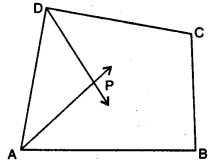Ans:
Here, AP and DP are angle bisectors of ∠A and ∠D
In ∆APD, ∠APD + ∠DAP + ∠ADP = 180°
⇒ ∠APD + 12 ∠DAB + 1/2∠ADC = 180°
⇒ ∠APD = 180° – 1/2(∠DAB + ∠ADC)
⇒ 2∠APD = 360° – (∠DAB + ∠ADC) ……(ii)
Also, ∠A + ∠B + C + ∠D = 360°
∠B + 2C = 360° – (∠A + ∠D)
∠B + C = 360° – (∠DAB + ∠ADC) ……(iii)
From (ii) and (iii), we obtain
2∠APD = ∠B + ∠C

Q2: In right triangle ABC, right-angled at C, M is the mid-point of hypotenuse AB. C is joined to M and produced to a point D such that DM = CM. Point D is joined to point B (see fig.). Show that : (i) ∆AMC ≅ ∆BMD (ii) ∠DBC = 90° (ii) ∆DBC ≅ ∆ACB (iv) CM = 1/2AB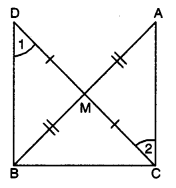Ans:

Given : ∆ACB in which 4C = 90° and M is the mid-point of AB.
To Prove :
(i) ∆AMC ≅ ∆BMD
(ii) ∠DBC = 90°
(iii) ∆DBC ≅ ∆ACB
(iv) CM = 1/2AB
Proof : Consider ∆AMC and ∆BMD,
we have AM = BM [given]
CM = DM [by construction]
∠AMC = ∠BMD [vertically opposite angles]
∴ ∆AMC ≅ ∆BMD [by SAS congruence axiom]
⇒ AC = DB …(i) [by c.p.c.t.]
and ∠1 = ∠2 [by c.p.c.t.]
But ∠1 and ∠2 are alternate angles.

⇒ BD || CA
Now, BD || CA and BC is transversal.
∴ ∠ACB + ∠CBD = 180°
⇒ 90° + CBD = 180°
⇒ ∠CBD = 90°
In ∆DBC and ∆ACB,
we have CB = BC [common]
DB = AC [using (i)]
∠CBD = ∠BCA
∴ ∆DBC ≅ ∆ACB
⇒ DC = AB
⇒ 1/2AB = 1/2DC
⇒ 1/2AB = CM or CM = 1/2AB (∵ CM = 1/2DC)

Q3: Prove that two triangles are congruent if any two angles and the included side of one triangle is equal to any two angles and the included side of the other triangle.
Ans: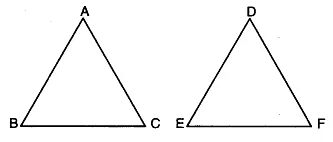Given : Two As ABC and DEF in which
∠B = ∠E,
∠C = ∠F and BC = EF
To Prove : ∆ABC = ∆DEF
Proof : We have three possibilities
Case I. If AB = DE,
we have AB = DE,
∠B = ∠E and BC = EF.
So, by SAS congruence axiom, we have ∆ABC ≅ ∆DEF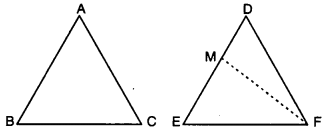Case II. If AB < ED, then take a point Mon ED
such that EM = AB.
Join MF.
Now, in ∆ABC and ∆MEF,
we have
AB = ME, ∠B = ∠E and BC = EF.
So, by SAS congruence axiom,
we have ΔΑΒC ≅ ΔΜEF
⇒ ∠ACB = ∠MFE
But ∠ACB = ∠DFE
∴ ∠MFE = ∠DFE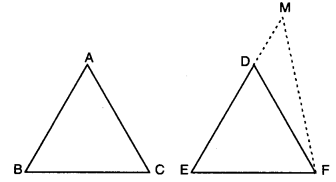Which is possible only when FM coincides with B FD i.e., M coincides with D.
Thus, AB = DE
∴ In ∆ABC and ∆DEF, we have
AB = DE,
∠B = ∠E and BC = EF
So, by SAS congruence axiom, we have
∆ABC ≅ ∆DEF
Case III. When AB > ED
Take a point M on ED produced
such that EM = AB.
Join MF
Proceeding as in Case II, we can prove that
∆ABC = ∆DEF
Hence, in all cases, we have
∆ABC = ∆DEF.

Q4: In the given figure, side QR is produced to the point S. If the bisectors of ∠PQR and ∠PRS meet at T, prove that ∠QTR = 1/2 ∠QPR.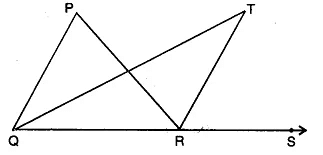Ans: Here, QT is angle bisector of ∠PQR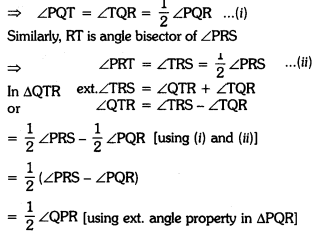Q5: In figure, ABC is an isosceles triangle with AB = AC. D is a point in the interior of ∆ABC such that ∠BCD = ∠CBD. Prove that AD bisects ∠BAC of ∆ABC.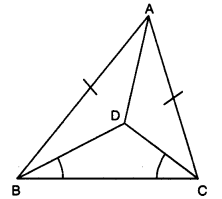Ans:
In ∆BDC, we have ∠DBC = ∠DCB (given).
⇒ CD = BD (sides opp. to equal ∠s of ∆DBC)
Now, in ∆ABD and ∆ACD,
we have AB = AC [given]
BD = CD [proved above]
∴ By using SSS congruence axiom, we obtain
∆ABD ≅ ∆ACD
Hence, AD bisects ∠BAC of ∆ABC.

Q6: In figure, ABCD is a square and EF is parallel to diagonal BD and EM = FM. Prove that (i) DF = BE (ii) AM bisects ∠BAD.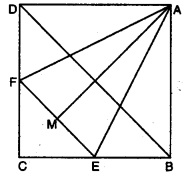Ans:
(i) EF || BD = ∠1 = ∠2 and ∠3 = ∠4 [corresponding ∠s]
Also, ∠2 = ∠4
⇒ ∠1 = ∠3
⇒ CE = CF (sides opp. to equals ∠s of a ∆]
∴ DF = BE [∵ BC – CE = CD – CF)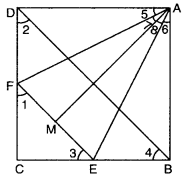(ii) In ∆ADF and ∆ABE, we have
AD = AB [sides of a square]
DF = BE [proved above]
∠D = ∠B = 90°
⇒ ∆ADF ≅ ∆ABE [by SAS congruence axiom]
⇒ AF = AE and ∠5 = ∠6 … (i) [c.p.c.t.]
In ∆AMF and ∆AME
AF = AE [proved above]
AM = AM [common]
FM = EM (given)
∴ ∆AMF ≅ ∆AME [by SSS congruence axiom]
∴ ∠7 = ∠8 …(ii) [c.p.c.t.]
Adding (i) and (ii), we have
∠5 + ∠7 = ∠6 + ∠8
∠DAM = ∠BAM

The document Long Question Answer: Triangles | Mathematics (Maths) Class 9 is a part of the Class 9 Course Mathematics (Maths) Class 9.
All you need of Class 9 at this link: Class 9

## Mathematics (Maths) Class 9

42 videos|378 docs|65 tests

## Mathematics (Maths) Class 9

42 videos|378 docs|65 testsExplore Courses for Class 9 examSignup to see your scores go up within 7 days! Learn & Practice with 1000+ FREE Notes, Videos & Tests.
10M+ students study on EduRev
Track your progress, build streaks, highlight & save important lessons and more!
Related Searches

,

,

,

,

,

,

,

,

,

,

,

,

,

,

,

,

,

,

,

,

,

;